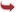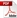International Journal of Scientific and Research Publications

#### IJSRP, Volume 3, Issue 9, September 2013 Edition [ISSN 2250-3153]A nonlinear mathematical model of the mammary carcinoma-immune system: An analytical approach
J. Xavier Adaikalaraj , L. Rajendran
Abstract: The mathematical modeling of the mammary carcinoma–immune system by an external stimulus is discussed. This model is based on a system of six ordinary differential equations which contains a nonlinear term related to various parameters and cell populations. Approximated closed analytical expressions for cell populations have been derived for all values of parameters for steady and non-steady state conditions. As time tends to infinity, the analytical expressions corresponding to the cell populations for non-steady state conditions approach the steady state values. These analytical results are of great interest both in the applied and theoretical sciences. Furthermore, in this work the numerical simulation of the problem is also reported using Matlab program.
[VIEW FULL PAPER][DOWNLOAD]

Reference this Research Paper (copy & paste below code):

J. Xavier Adaikalaraj , L. Rajendran (2018); A nonlinear mathematical model of the mammary carcinoma-immune system: An analytical approach; Int J Sci Res Publ 3(9) (ISSN: 2250-3153). http://www.ijsrp.org/research-paper-0913.php?rp=P211699